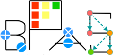Basic Polynomial Algebra Subprograms (BPAS)  v. 1.652
BPASEuclideanDomain.hpp
1
2 #ifndef _BPAS_EUCLIDEAN_DOMAIN_H_
3 #define _BPAS_EUCLIDEAN_DOMAIN_H_
4
5 #include "BPASGCDDomain.hpp"
6 #include "../Utils/TemplateHelpers.hpp"
7
8 /**
9  * An abstract class defining the interface of a Euclidean domain.
10  */
11 template <class Derived>
12 class BPASEuclideanDomain : public virtual BPASGCDDomain<Derived> {
13
14 public:
15
16  /**
17  * Get the euclidean size of *this.
18  */
19  virtual Derived euclideanSize() const = 0;
20
21  /**
22  * Perform the eucldiean division of *this and b. Returns the
23  * remainder. If q is not NULL, then returns the quotient in q.
24  */
25  virtual Derived euclideanDivision(const Derived& b, Derived* q = NULL) const = 0;
26
27  /**
28  * Perofrm the extended euclidean division on *this and b.
29  * Returns the GCD. If s and t are not NULL, returns the bezout coefficients in them.
30  */
31  virtual Derived extendedEuclidean(const Derived& b, Derived* s = NULL, Derived* t = NULL) const = 0;
32
33  /**
34  * Get the quotient of *this and b.
35  */
36  virtual Derived quotient(const Derived& b) const = 0;
37
38  /**
39  * Get the remainder of *this and b.
40  */
41  virtual Derived remainder(const Derived& b) const = 0;
42
43  /**
44  * Get the remainder of *this and b;
45  */
46  virtual Derived operator%(const Derived& b) const = 0;
47
48  /**
49  * Assign *this to be the remainder of *this and b.
50  */
51  virtual Derived& operator%=(const Derived& b) = 0;
52
53
54 };
55
56 #endif
An abstract class defining the interface of a GCD domain.
Definition: BPASGCDDomain.hpp:14
virtual Derived operator%(const Derived &b) const =0
Get the remainder of *this and b;.
virtual Derived & operator%=(const Derived &b)=0
Assign *this to be the remainder of *this and b.
virtual Derived euclideanSize() const =0
Get the euclidean size of *this.
virtual Derived extendedEuclidean(const Derived &b, Derived *s=NULL, Derived *t=NULL) const =0
Perofrm the extended euclidean division on *this and b.
virtual Derived remainder(const Derived &b) const =0
Get the remainder of *this and b.
An abstract class defining the interface of a Euclidean domain.
Definition: BPASEuclideanDomain.hpp:12
virtual Derived quotient(const Derived &b) const =0
Get the quotient of *this and b.
virtual Derived euclideanDivision(const Derived &b, Derived *q=NULL) const =0
Perform the eucldiean division of *this and b.